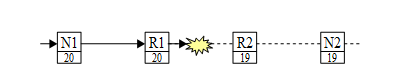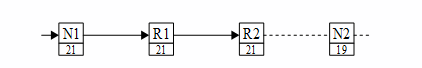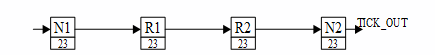If a received time-code is not one more than (modulo 64) the current time-count at the receiving link-interface, then either the time-code or the time-count shall be considered invalid. This can happen if a time-code is lost, or if a link is reset or restarted after a disconnect.

If the time-code is invalid then the time-count is updated to the new value but the time-code is not propagated in a router and TICK_OUT is not asserted in a node. This prevents propagation of invalid time-codes across a network. When the next time-code is received it is expected that the time-counter matches the time-code and normal operation resumes. Recovery from missing or invalid time-codes will now be considered.

Figure 73 shows a SpaceWire network in which a time-code with a time-value of 20 is lost between R1 and R2.Figure 73 Lost Time-Code

On the next tick N1 sends out the time-code 21. R1 then forwards this time-code to R2. This is not same as, nor one more than time-counter of R2 so R2 updates its time-counter but does not emit the time-code, as shown in Figure 74.Figure 74 R2 Time-Counter Updated

On the next tick the time-code 22 is sent from N1 to R1, which forwards it on to R2. At R2 the time-count is now 21, so the incoming time-code is one more than the time-count hence the time-code is now valid and is propagated by the router and reaches N2. See Figure 75. When the time-code reaches N2 it is not one more than N2’s time-count (value 19) so the time-code is deemed invalid. N2 updates its time count to 22 but does not give a TICK_OUT.Figure 75 N2 Time-Counter Updated

The next tick will result in the time-code 23 propagating across the network and N2 will produce a TICK_OUT, as shown in Figure 76.Figure 76 N2 Gets Valid Time-Code

It takes several ticks to recover from initial error, depending upon the size of the network.

Note that if there is an alternative path from R1 to R2 the time-code may propagate successfully through the alternative path so that R2 gets a valid time-code even though one of the time-codes on its way to R2 gets lost. This provides a first level of fault tolerance for time-code distribution.

Nodes using the time-code distribution function can either use the TICK_OUT signal as a periodic timing signal or use the value of the time-count as an indication of the least-significant 6-bits of system time.

As a missing tick results in a timing discrepancy, the TICK_OUT signal should not be used to increment a counter with the expectation that this counter always corresponds to the system time. Rather a time-lock technique should be used where a free running local time-counter is updated to be an exact multiple of the system tick rate every time the TICK_OUT signal is asserted. The reason for this is that when using the TICK_OUT signal as a periodic timing signal the time-code can be missed so that a TICK_OUT signal is missed. Having said this, SpaceWire signals running over 10m SpaceWire cable have a good eye diagram and are unlikely to give rise to any errors.